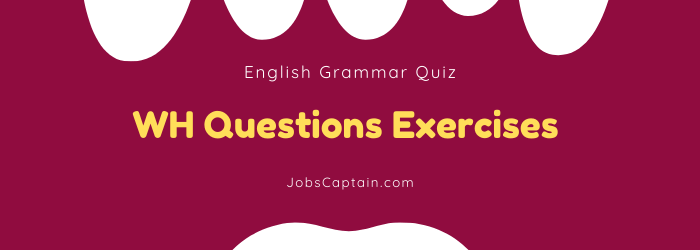# Latest WH Questions Exercises With AnswerWith the WH questions exercises that you can use to improve your English conversation skills. Check out WH questions that will help you practice using who, what, when, where, why, and how.

This blog post contains a quiz on WH questions for you to test your knowledge as well as some exercises to practice. By the end of this post, you will be an expert on WH questions!

## WH Types Questions Exercises

Question 1: ________ milk is there in the bottle?

(A) How many

(B) How far

(C) How much

(D) How long

(C) How much

Question 2: ________ of the girls is the tallest, Meera or Ritu?

(A) Which

(B) who

(C) whom

(D) What

(A) Which

Question 3: ________ do you think is the best cricketer now?

(A) Which

(B) Who

(C) Whom

(D) What

(B) Who

Question 4: ________ of you work for cleanliness every day?

(A) How much

(B) How long

(C) How

(D) How many

(D) How many

Question 5: ________ beautiful this place is!

(A) How

(B) What

(C) Who

(D) Why

(A) How

Question 6: ________ milk is there in the cup?

(A) How long

(B) How far

(C) How many

(D) How much

(D) How much

Question 7: ________ of these books is your favorite one?

(A) Which

(B) Why

(C) Whose

(D) What

(A) Which

Question 8: ________ is your pen, red or blue?

(A) which

(B) who

(C) whom

(D) whose

(A) which

Question 9: ________ did you live in Himatnagar?

(A) Where

(B) Who

(C) Which

(D) What

(A) Where

Question 10: ________ sugar is there in the bowl?

(A) How many

(B) How much

(C) How far

(D) None

(B) How much

Question 11: ________ is your father? Has he recovered fully?

(A) How

(B) Who

(C) What

(D) When

(A) How

Question 12: ________ will you meet if the Boss is not available?

(A) Who

(B) Whom

(C) Whose

(D) Which

(B) Whom

Question 13: ________ do you visit the library in a week?

(A) How often

(B) How much

(C) How far

(D) How long

(A) How often

Question 14: ________ money do you spend on books?

(A) How

(B) How often

(C) How many

(D) How much

(D) How much

Question 15: ________ among you have ________ visited the Sinhagadh?

(A) How, ever

(B) Which, never

(C) Whom, be

(D) Who, ever

(B) Which, never

Question 16: ________ do you visit the library in a week?

(A) Where

(B) How far

(C) How often

(D) However

(C) How often

Question 17: ________ will it take to reach the project site?

(A) However

(B) How long

(C) How many

(D) How often

(B) How long

Question 18: ________ did you pay for your new car?

(A) However

(B) How

(C) How many

(D) How much

(D) How much

Question 19: ________ do you visit the library in a week?

(A) How is

(B) How often

(C) How much

(D) How many

(B) How often

Question 20: ________ did you stay in Ambaji?

(A) However

(C) How many

(D) How long

(D) How long

Question 21: ________ is Mathura from Kashi?

(A) How much

(B) How far

(C) How many

(D) How often

(B) How far

Question 22: ________ water is left in the tank?

(A) How much

(B) How long

(C) How far

(D) How often

(A) How much

Question 23: Since ________ have you been waiting for her?

(A) however

(B) how much

(C) how many

(D) how long

(D) how long

Question 24: When are you expected to be vigorous?

(A) While sleeping

(B) When you are hungry

(C) While doing exercise

(D) When you are waiting

(C) While doing exercise

Question 25: ________ do you think, will get the first prize?

(A) Who

(B) Whom

(C) whose

(D) How

(A) Who

Question 26: The king set three difficult tests for princes.

_______ tests did the king set for princes? Select proper question word.

(A) How much

(B) How

(C) How many

(D) Who

(C) How many

Question 27: “________ is the dog hiding?” “It’s behind the tree.”

(A) When

(B) Where

(C) Why

(D) How

(B) Where

Question 28: “________ will you come to our house?” “After three days.”

(A) When

(B) Where

(C) Why

(D) How

(A) When

Question 29: “________ do we eat?” “We eat to survive/live.”

(A) When

(B) Where

(C) Why

(D) How

(C) Why

Question 30: How ________ children have you?

(A) much

(B) more

(C) many

(D) long

(C) many

Question 31: “________ feels thirsty? ” “Jiya.”

(A) Who

(B) Whose

(C) Which

(D) Where

(A) Who

Question 32: ________ did the family members go yesterday?

(A) Why

(B) where

(C) When

(D) Whose

(B) where

Question 33: ________is the poem being read?

(A) By whom

(B) Who

(D) Whose

(A) By whom

Question 34: “________ boys are there?” “There are two boys.”

(A) How much

(B) How many

(C) Why

(D) Which

(B) How many

Question 35: “________ are you today?” “I’m fine, thank you.”

(A) How

(B) Who

(C) What

(D) Where

(A) How

Question 36: ________ is the minister absent today?

(A) Why

(B) When

(C) Who

(D) Whom

(A) Why

Question 37: ________ plays badminton every Sunday?

(A) Who

(B) Whom

(C) She

(D) What

(A) Who

Question 38: ________ plays badminton every Sunday.

(A) Who

(B) Whom

(C) She

(D) What

(C) She

Question 39: How ________does this T.V cost?

(A) many

(B) often

(C) much

(D) far

(C) much

Question 40: ________ Colours are in our flag?

(A) What

(B) How many

(C) How

(D) Whose